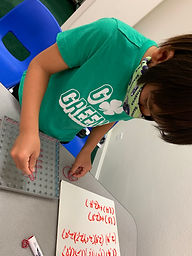Ms. Kayla

Target 1​

Lesson Type:

Review

Number Operation

:

Number Reltionships

Compare numbers, recording the results of the comparison with the symbol >, =, and <.

1:

Understand how to use place-value (the value of each digit) to compare numbers.

2:

When comparing decimals, understand to look at the greatest place-value first.

3:

Explain how a number could have a lesser value, even if it has more digits than another (i.e., 4.33<5.2).

5th

Vocabulary:

Compare, Greater than, Less than, Equal, Decimal, Multiply, Add, Subtract,

Activities:

1. Students were given multiple number to compare.

2. Students used symbols to show greater than, less than, or equal to.Home Exploration

Guiding Questions:Absent Students:

Target 2

:

1:

Identify the x- and y-axis on a coordinate plane.

2:

Understand that the first number in a coordinate pair indicates placement on the x-axis and the second indicates placement on the y-axis.

3:

Graph points in the first and second quadrant of the coordinate plane.

4th

Vocabulary:

Coordinate, Coordinate Pairs, Grid/Plane, Quadrilateral, X-Axis, Y-Axis

Activities:

1. Students used geoboards to plot coordinate pairs and to construct geometric shapes (quadrilaterals).

2. Students located points on their coordinate grid.

3. Students constructed geometric shapes on their geoboards and played Battleship. Students said coordinate pairs to "fire" and try to sink the other's battleship.Home Exploration

Guiding Questions:Target 3

:

1:

Accurately measure angles.

2:

Identify angles as acute, obtuse, or right.

3:

Identify angle types and estimate their measurement.

4:

Understand that angles are measured in degrees (from 0 to 360).

5:

Understand that angles have types based on their degree measures (acute, obtuse, and right).

5th

Vocabulary:

Angle, Acute, Obtuse, Right, Straight angle, Protractor, Triangle, Rectangle, Circle, Degree

Activities:

1. Students used a protractor to draw angles. were introduced to the protractor that was usewere inwere were introduced to the protractor thatw as used to create aswere introduced tothe protractor to create specific anused a protractor to create specific angles.

useusess

useddd

used pratotractors to create specific anglesStudents were able to identify an angle either acute, obtuse, or right.

2. Students identified angles as either acute, obtuse, or right angles.

3. Students were also introduced to the straight angle.

4. Students created triangles, rectangles, and circles to determine the sum of the angle.Home Exploration